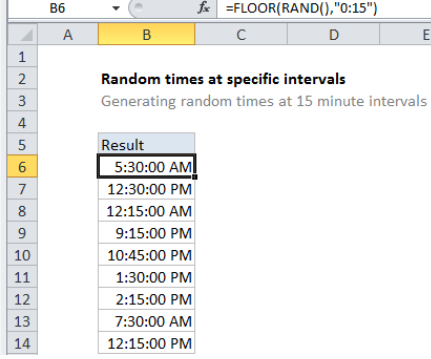# How to generate random times at specific intervals in Excel

To generate random times in at specific intervals you can use the RAND function with the FLOOR function. See example below:

## Formula

`=FLOOR(RAND(),"0:15")`## Explanation

In the example shown, the formula in B6 is:

`=FLOOR(RAND(),"0:15")`

which generates a random time at a 15-minute interval.

### How this formula works

The RAND function generates a decimal number between zero and 1. So, you might get output like this from RAND() in three cells:

Worked Example:   How to fill cell ranges with random text values in Excel

0.54739314
0.919767722
0.633760119

Dates in Excel are defined as simple numbers, where 1 = 1 day. This means you can simply divide 1 by the decimal value of time to get a value that corresponds to time as Excel sees it, for example:

1/12 = 12 hours = .5 days
1/6 = 6 hours = .25 days
1/8 = 8 hours = .333 days

This means we can use RAND() to generate a decimal value between 1 and 0, then round that number down with FLOOR to the nearest interval. FLOOR takes a argument called “significance” as the rounding multiple, and it recognizes time intervals expressed like this:

Worked Example:   How to get random value from list or table in Excel

“0:10” – 15 minutes
“0:15” – 10 minutes
“0:30” – 30 minutes
“0:45” – 45 minutes

### Setting and upper and lower time

If you want to limit the hours used by RAND, you can use this general formula force RAND to output a number between an upper and lower value:

`=RAND()*(upper-lower)+lower`

Because Excel can recognize time values, you can get times between 8 AM and 12 PM, with a formula like this:

`=RAND()*("12:00"-"8:00")+"8:00"`

Note: the formula above is general and will work with other numbers too, not just times.